GeeksforGeeks App
Open AppBrowser
Continue

# Slope-Intercept Form of Straight Lines

Straight lines can be viewed as a point extended indefinitely in any two opposite directions. A straight line is one that has no curves and covers an infinite number of points.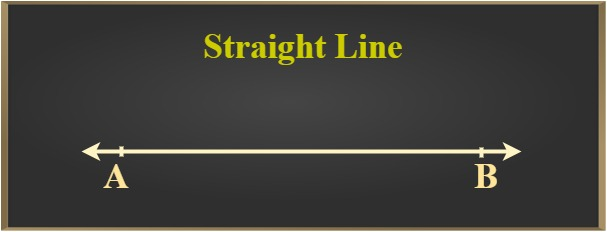### Properties of a straight line

• Infinite number of straight lines pass through a point
• Infinite numbers of planes contain a straight line (when viewed in 3-Dimensions)
• A straight line has infinite number of points
• Line segment joining any two points on a straight line lies on the straight line

### Basic Terminologies

• Point: A point is a dimensionless geometrical figure which has a location (with respect to some reference e.g: points in a coordinate system).
• Plane: A flat surface of the infinite area. It is 2-Dimensional.
• Ray: A point when extended indefinitely in any one direction in a plane forms a ray.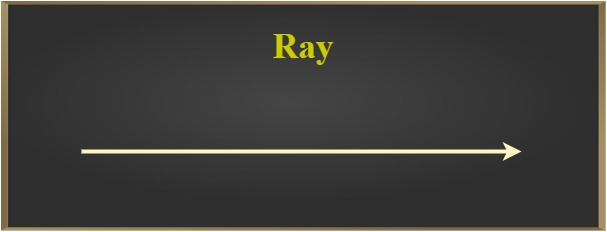• Line segment: The shortest curve joining two points is called a line segment.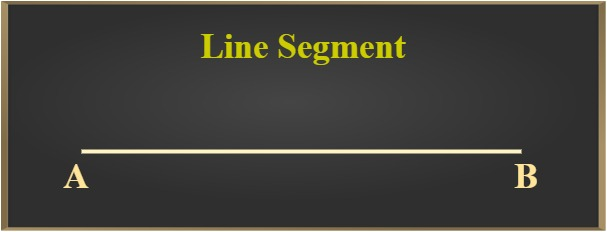Straight lines are studied in 2-D and 3-D. We are going to discuss straight lines in 2-D and for this purpose, we use the coordinate system.

### Coordinate axes

Two perpendicular lines which divide the plane (of our consideration) into 4 regions called quadrants.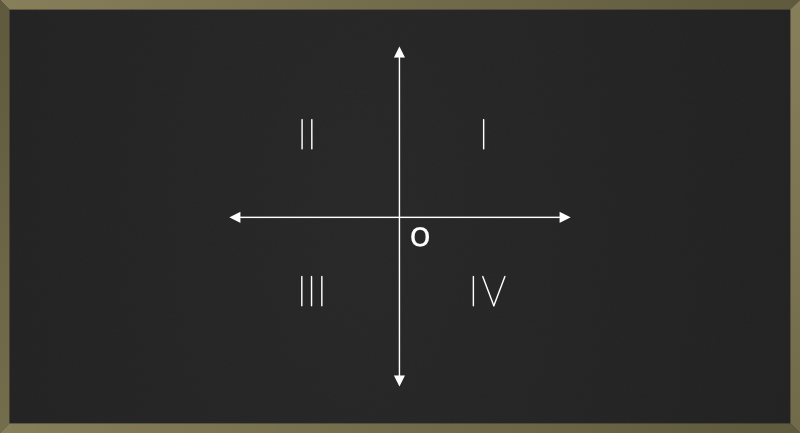Let AB be a straight line in this plane. Let the angle between line AB and the X-axis be w°. Then line AB can be:

• Parallel to X-axis, w = 0°
• Parallel to Y-axis, w = 90°
• At an angle w° with the X-axis (see Fig. below), 0° < w° < 180°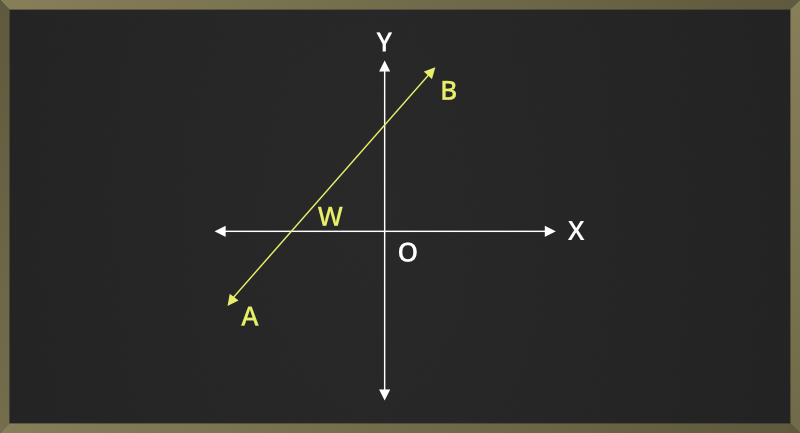### Slope of a Straight Line

It is defined as the tangent of the angle (here tan(w)) between the line and the X-axis as shown below: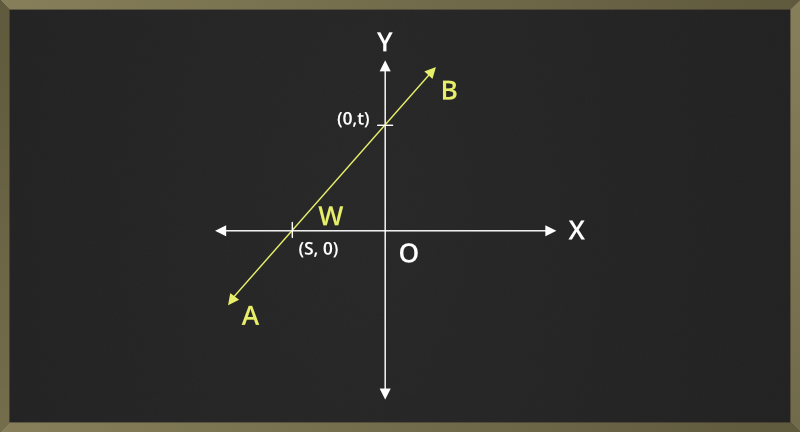slope of line AB

= tan(w)

= (0 – t) / (s – 0)

= t/s

i.e. if (x1, y1) and (x2, y2) are two points on line AB then,

slope of line AB

= tan(w)

= (y2 – y1) / (x2 – x1)

= (y1 – y2) / (x1 – x2)

= distance between the y coordinate of both points / distance between the x coordinate of both points

### X-intercept

The point of intersection of a line and the x-axis is called the x-intercept.

Example: Here, s is the x-intercept of line AB### Y-intercept

The point of intersection of a line and the y-axis is called the y-intercept.

Example: Here, t is the y-intercept of line AB### Equations Of Straight Lines

Straight lines can be represented in various forms:

• Slope-intercept form
• Point-slope form
• Two-point form, etc.

We are going to discuss (i) slope-intercept from keeping the above figure in mind.

Equation of the straight line in slope-intercept form is:

y = slope * x + c

y = tan(w) * x + c

y = mx + c

Here m = tan(w) = slope of line AB and c is the y-intercept.

### Sample Problems on Straight Lines

Now, we can easily do the following tasks:

Problem 1: Write the equation of a straight line whose slope is p and y-intercept is g.

Solution: y = p * x + g

Problem 2: Write the equation of a straight line whose slope is 1/2 and y-intercept is 11.

Solution: y = (1/2) * x + 11

Problem 3: Write the equation of a straight line that passes through the origin and has slope 1/6.

Solution:

Since the line passes through the origin,

Y-intercept = 0

y = (1/6) * x + 0

y = (1/6)x,

which is the required equation.

Problem 4: Find the slope and y-intercept of the line represented by the equation y = tx + 4

Solution:

The equation of the given line is in slope-intercept form.

Thus, slope of the given line is t and its y-intercept is 4.

Problem 5: Find the slope and y-intercept of the line represented by the equation y + 4 = x

Solution:

The given equation is not in slope-intercept form.

Therefore, we convert it to that form.

y = x – 4

y = 1 * x – 4

Thus, slope of the given line is 1 and its y-intercept is -4.

Problem 6: Find the slope and y-intercept of the line represented by the equation x = y/q

Solution:

The given equation is not in slope-intercept form.

Therefore, we convert it to that form.

y = qx

y = qx + 0

Thus, slope of the given line is q and its y-intercept is 0.

Problem 7: Find the slope and y-intercept of the line represented by the equation x = d

Solution:

The given equation is not in slope-intercept form.

Moreover, the variable y is not present in the equation i.e. the equation of this line is independent of the value of variable y.

Equations like this represent lines which are parallel to y-axis.

For lines that are parallel to y-axis w = 90°,

thus the slope of these lines is not defined because tan(90°) is not defined.

Since, the line is parallel to y-axis, it never intersects the y-axis and hence does not have y-intercept.

Problem 8: Find the slope and y-intercept of the line represented by the equation y = h

Solution:

The given equation is not in slope-intercept form.

Moreover, the variable x is not present in the equation i.e.

the equation of this line is independent of the value of variable x.

Equations like this represent lines which are parallel to x-axis.

Given equation can be rewritten as:

y = 0x + h,
which is the slope intercept form of the given line.

Thus, slope of given line is 0 and its y-intercept is h.

### Sample Word Problems

Problem 1: The base fare for riding a taxi is Rs 30.00 for the first 2 km. After, this the rider is charged Rs 4 per km. Calculate the fare for traveling the distance of 14 km by forming a linear equation.

Solution:

Base fare = Rs 30, this means that Rs 30 is the minimum fare.

Therefore, the fare for the distances from 1m to 2000m (2km) is Rs 30.

Fare for distances after 2 km is Rs. 4/km.

Let the total distance traveled be d km and total cost be Rs c.

Charge up to the first 2 km charge is Rs 30.00.

Charge for the rest of the (d-2) km charge is Rs 4 * (d – 2).

Therefore, total fare is given by the equation,

c = 4 * (d – 2) + 30

c = 4 * d – 8 + 30

c = 4 * d + 22,

(which is of the slope intercept form)

Given, d = 14km,

Therefore, total cost,

c = 4 * 14 + 22

= 56 + 22

= 78

Thus, the fare for travelling the distance of 14km is Rs 78.

Problem 2: The base fare for riding a taxi is Rs 30.00 for the first 2 km. After, this the rider is charged Rs 4 per 600m. Calculate the fare for traveling the distance of 14 km by forming a linear equation.

Solution:

Base fare = Rs 30, this means that Rs 30 is the minimum fare.

Therefore, the fare for the distances 1m to 2000m (2km) is Rs 30.

Fare for distances after 2 km is Rs4 per 600m.

Let the total distance traveled be d km and total cost be Rs c.

Up to the first 2 km charge is Rs 30.00.

Remaining distance = (d – 2)km

Charge for 600 m = Rs 4

Charge for 1 m = Rs (4/600)         ———(unitary method)

Charge for 1000m = Rs. (4 / 600) * 1000 = Rs (40/6)

Therefore, the charge per km after the first 2 km is Rs (40/6) per km.

Charge for the rest of the (d – 2) km is Rs (40/6) * (d – 2).

Therefore, total fare is given by the equation,

c = (40/6) * (d – 2) + 30

c = (40/6) * d – 20/6 + 30

c = (20/3) * d + (-20 + 180) / 6

c = (20/3) * d + (160/6)

c = (20/3) * d + (80/3),

(which is of the slope-intercept form)

Given, d = 14km,

Therefore, total cost,

c = (20/3) * 14 + (80/3)

= (280/3) + (80/3)

= 360/3

= 120 (approx.)

Thus, the total fare for traveling the distance of 14km is Rs 120 (approximately).

Problem 3: Plane number 13 owned by Earth Airlines is undergoing repair. The engineer is informed that the airlines could suffer a loss of Rs 1300 per 12 minutes until it is repaired. The engineer was told that the airlines would not suffer a loss greater than Rs5000 and that the extra amount would be deducted from his salary. Calculate

• The maximum time in minutes within which the engineer has to repair the airplane.
• The total time taken if the total loss is Rs 6000

Solution:

Loss in 12 min = Rs. 1300

Loss in 1 min = Rs. (1300/12) = Rs. (325/3)

Therefore, loss per minute is Rs. (325/3).

(i)
Let the maximum time be t minutes.

Therefore, t * (325/3) = 5000

or t = (5000 * 3)/325

or t = 46.15 minutes = 46 minutes (approx.)

Thus, the engineer has to repair the airplane within 46 minutes.

(ii)
Total loss = Rs 6000 (Given)

Let the time taken for this loss be k minutes.

Time required for loss of Rs(325/3) = 1 min  (unitary method)

Time required for loss of Rs 1 = 1 * (3/325)min

Therefore, time required for loss of Rs 6000 = 6000 * (3/325) minutes = 55.384 minutes

Problem 4: X and y are two 2-digit numbers which satisfy the following conditions:

• y > x
• x + y = m, where m is the 2-digit number obtained by reversing the digits of y
• y  – x = x – 1
• Tens digit of y – Units digit of y = -2

Find the two numbers.

Solution:

y – x = x – 1,       ——-condition(iii) can be rewritten as

y = x + x – 1

y = 2x – 1       ———-(1)

Let the tens and units digits of the number y be a and b respectively.

This means that y = 10a + b

and m = 10b + a

From condition(ii),

x + y = m

x + 10a + b = 10b + a

9a – 9b + x = 0

9(a – b) + x = 0

9 * (-2) + x = 0, ——-condition (iv)

-18 + x = 0

Therefore, x = 18

Substituting the value of x in equation (1),

y = 2 * 18 – 1

y = 36 – 1

Therefore, y = 35

Problem 5: The sum of the scores of two friends in a subject is 100.  The difference of their scores in that same subject is 10. Find their scores.

Solution:

Let the scores be x and y such that x > y.

Given, x + y = 100 —-(1)

and    x – y = 10  —-(2)

x + y + x – y  = 100 + 10

2x + 0y = 110

2x = 110

x = 110/2 = 55

Substituting value of x in equation (2)

55 – y = 10

55 – 10 = y

y = 45

Therefore, their scores are 45 and 55.

My Personal Notes arrow_drop_up
Related Tutorials